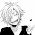## J.M.'s Proportion Problem

http://bashooka.com/inspiration/creative-poster-designs/

1) Three posters cost \$ 9.60. At that rate, how many posters can you buy for \$ 48?

Let x be how many posters can be bought with \$48
Steps, Word Ratio, Ratio Table

Poster| \$
3    | 9.60                                                     9.60x = 3
x   | 48                                                        9.60x = 144
9.60       9.60
x = 15

I can buy 15 posters with \$48.

2) You estimate that you can do 12 math problems in 45 minutes. How long should it take you to do 20 math problems?

Let x be the minutes it should take me to do 20 math problems
Steps Word Ratio, Ratio Table

Minutes | Math Problems
45     |    12                                                     12x = 45
x      |     20                                                    12x = 900
12       12
x = 75

It should take me 75 minutes for me to do 20 math problems

Which cost more per issue, an 18 - issue subscription for \$40. 50 or a 12 - issue subscription for \$33.60?

3) Let x be
Steps, Word Ratio, Ratio Table

Subscription | \$
18         | 40. 50
12         | 33.60

5:38 AM

#### 1 comment :

1.J.M. one question do we have to answer our own question or other answer our question?